# 28 Results

View
Selected filters:
• Order of OperationsConditional Remix & Share Permitted
CC BY-NC-SA
Rating

This short video and interactive assessment activity is designed to teach fifth graders about adding, subtracting, multiplying and dividing.

Subject:
Math
Foundations
Material Type:
Activity/Lab
Lesson
Provider:
CK-12 Foundation
Provider Set:
CK-12 Elementary Math
05/03/2018Conditional Remix & Share Permitted
CC BY-NC-SA
Rating

This short video and interactive assessment activity is designed to teach fifth graders about adding, subtracting, multiplying, and dividing with parentheses.

Subject:
Math
Foundations
Material Type:
Activity/Lab
Lesson
Provider:
CK-12 Foundation
Provider Set:
CK-12 Elementary Math
05/03/2018Conditional Remix & Share Permitted
CC BY-NC-SA
Rating

This short video and interactive assessment activity is designed to teach fifth graders about adding, subtracting, and multiplying.

Subject:
Math
Foundations
Material Type:
Activity/Lab
Lesson
Provider:
CK-12 Foundation
Provider Set:
CK-12 Elementary Math
05/03/2018Conditional Remix & Share Permitted
CC BY-NC-SA
Rating

This short video and interactive assessment activity is designed to teach fifth graders about adding and subtracting.

Subject:
Math
Foundations
Material Type:
Activity/Lab
Lesson
Provider:
CK-12 Foundation
Provider Set:
CK-12 Elementary Math
05/03/2018Conditional Remix & Share Permitted
CC BY-NC-SA
Rating

This short video and interactive assessment activity is designed to teach fifth graders about adding and subtracting with parentheses.

Subject:
Math
Foundations
Material Type:
Activity/Lab
Lesson
Provider:
CK-12 Foundation
Provider Set:
CK-12 Elementary Math
05/03/2018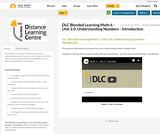Conditional Remix & Share Permitted
CC BY-NC-SA
Rating

The purpose of this lesson is to introduce the unit on understanding numbers in grade 6 math.

Included is a YouTube video to support Grade 6 Blended Learning Math - Unit 2.0: Understanding Numbers - Introduction.

Subject:
Math
Material Type:
Activity/Lab
Homework/Assignment
Lesson
Provider:
Sun West Distance Learning Centre (DLC)
Author:
Sun West School Division
05/10/2019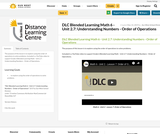Conditional Remix & Share Permitted
CC BY-NC-SA
Rating

The purpose of this lesson is to explore using the order of operations to solve problems.

Included is a YouTube video to support Grade 6 Blended Learning Math - Unit 2.7: Understanding Numbers - Order of Operations.

Subject:
Math
Material Type:
Activity/Lab
Homework/Assignment
Lesson
Provider:
Sun West Distance Learning Centre (DLC)
Author:
Sun West School Division
05/14/2019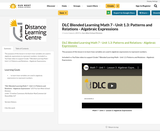Conditional Remix & Share Permitted
CC BY-NC-SA
Rating

The purpose of this lesson is to learn how variables are used in algebraic expressions to represent numbers.

Included is a YouTube video to support Grade 7 Blended Learning Math - Unit 1.3: Patterns and Relations - Algebraic Expressions.

Subject:
Math
Material Type:
Activity/Lab
Homework/Assignment
Lesson
Provider:
Sun West Distance Learning Centre (DLC)
Author:
Sun West School Division
06/06/2019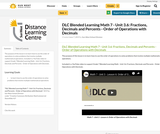Conditional Remix & Share Permitted
CC BY-NC-SA
Rating

The purpose of this lesson is to learn how to use the order of operations to solve problems that involve multiple mathematical operations.

Included is a YouTube video to support Grade 7 Blended Learning Math - Unit 3.6: Fractions, Decimals and Percents - Order of Operations with Decimals.

Subject:
Math
Material Type:
Activity/Lab
Homework/Assignment
Lesson
Provider:
Sun West Distance Learning Centre (DLC)
Author:
Sun West School Division
06/07/2019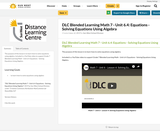Conditional Remix & Share Permitted
CC BY-NC-SA
Rating

The purpose of this lesson is to learn how to solve equations using algebra.

Included is a YouTube video to support Grade 7 Blended Learning Math - Unit 6.4: Equations - Solving Equations Using Algebra.

Subject:
Math
Material Type:
Activity/Lab
Homework/Assignment
Lesson
Provider:
Sun West Distance Learning Centre (DLC)
Author:
Sun West School Division
06/12/2019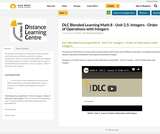Conditional Remix & Share Permitted
CC BY-NC-SA
Rating

The purpose of this lesson is to learn how to solve problems that include several different operations, including multiplication, division, subtraction and addition with negative and positive integers.

Included is a YouTube video to support Grade 8 Blended Learning Math - Unit 2.5: Integers - Order of Operations with Integers.

Subject:
Math
Material Type:
Activity/Lab
Homework/Assignment
Lesson
Provider:
Sun West Distance Learning Centre (DLC)
Author:
Sun West School Division
06/14/2019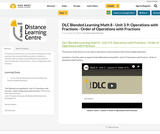Conditional Remix & Share Permitted
CC BY-NC-SA
Rating

The purpose of this lesson is to use order of operations to solve questions that involve multiple operations.

Included is a YouTube video to support Grade 8 Blended Learning Math - Unit 3.9: Operations with Fractions - Order of Operations with Fractions.

Subject:
Math
Material Type:
Activity/Lab
Homework/Assignment
Lesson
Provider:
Sun West Distance Learning Centre (DLC)
Author:
Sun West School Division
06/14/2019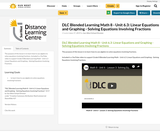Conditional Remix & Share Permitted
CC BY-NC-SA
Rating

The purpose of this lesson is to learn how to use algebra to solve equations involving fractions.

Included is a YouTube video to support Grade 8 Blended Learning Math - Unit 6.3: Linear Equations and Graphing - Solving Equations Involving Fractions.

Subject:
Math
Material Type:
Activity/Lab
Homework/Assignment
Lesson
Provider:
Sun West Distance Learning Centre (DLC)
Author:
Sun West School Division
06/17/2019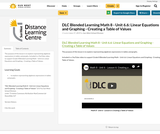Conditional Remix & Share Permitted
CC BY-NC-SA
Rating

The purpose of this lesson is to explore representing algebraic expressions in tables and graphs.

Included is a YouTube video to support Grade 8 Blended Learning Math - Unit 6.6: Linear Equations and Graphing - Creating a Table of Values.

Subject:
Math
Material Type:
Activity/Lab
Homework/Assignment
Lesson
Provider:
Sun West Distance Learning Centre (DLC)
Author:
Sun West School Division
06/17/2019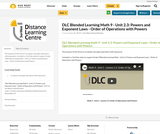Conditional Remix & Share Permitted
CC BY-NC-SA
Rating

The purpose of this lesson is to explain and apply operations with exponents.

Included is a YouTube video to support Grade 9 Blended Learning Math - Unit 2.3: Powers and Exponent Laws - Order of Operations with Powers.

Subject:
Math
Material Type:
Activity/Lab
Homework/Assignment
Lesson
Provider:
Sun West Distance Learning Centre (DLC)
Author:
Sun West School Division
06/18/2019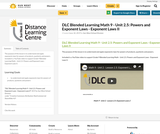Conditional Remix & Share Permitted
CC BY-NC-SA
Rating

The purpose of this lesson is to understand and apply exponents laws for powers of products, quotients and powers.

Included is a YouTube video to support Grade 9 Blended Learning Math - Unit 2.5: Powers and Exponent Laws - Exponent Laws II.

Subject:
Math
Material Type:
Activity/Lab
Homework/Assignment
Lesson
Provider:
Sun West Distance Learning Centre (DLC)
Author:
Sun West School Division
06/18/2019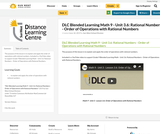Conditional Remix & Share Permitted
CC BY-NC-SA
Rating

The purpose of this lesson is to explain and apply the order of operations with rational numbers.

Included is a YouTube video to support Grade 9 Blended Learning Math - Unit 3.6: Rational Numbers - Order of Operations with Rational Numbers.

Subject:
Math
Material Type:
Activity/Lab
Homework/Assignment
Lesson
Provider:
Sun West Distance Learning Centre (DLC)
Author:
Sun West School Division
06/18/2019Conditional Remix & Share Permitted
CC BY-NC-SA
Rating

This short video and interactive assessment activity is designed to teach fifth graders about adding and subtracting - missing operation.

Subject:
Math
Foundations
Material Type:
Activity/Lab
Lesson
Provider:
CK-12 Foundation
Provider Set:
CK-12 Elementary Math
05/03/2018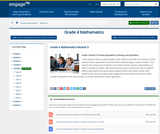Conditional Remix & Share Permitted
CC BY-NC-SA
Rating

In this 40-day module, students build on their Grade 3 work with unit fractions as they explore fraction equivalence and extend this understanding to mixed numbers.  This leads to the comparison of fractions and mixed numbers and the representation of both in a variety of models.  Benchmark fractions play an important part in students ability to generalize and reason about relative fraction and mixed number sizes.  Students then have the opportunity to apply what they know to be true for whole number operations to the new concepts of fraction and mixed number operations.

Subject:
Math
Material Type:
Module
Provider:
New York State Education Department
Provider Set:
EngageNY
01/17/2014Conditional Remix & Share Permitted
CC BY-NC-SA
Rating

This short video and interactive assessment activity is designed to teach fifth graders about multiplying and dividing.

Subject:
Math
Foundations
Material Type:
Activity/Lab
Lesson
Provider:
CK-12 Foundation
Provider Set:
CK-12 Elementary Math